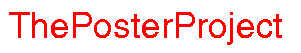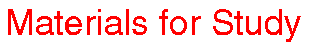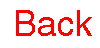Visualizing Women in Science, Mathematics and Engineering
• Home
• Posters
• Materials for Study
• Biographies
•Sometimes all we know about a given system is its rate of change. Let me give you a concrete example. Suppose you're interested in knowing how much water is behind the Example Dam. You stand on the mighty dam walls and watch the water rush out of a special gate. This really is a big dam. You reckon 100 gallons rush out every second. Can you use this information to work out how much water is in the dam at any given time? Suppose we write the amount of water in the dam at time t by W(t). (If this notation scares you, you should read about functions on the analysis page.) All we know is the rate of change of W(t) -- we know that it decreases by 100 gallons per second. We write this as W '(t)=-100. The minus sign is because the amount of water behind the dam is decreasing. So we've abstracted the problem to the following: Can we find W(t) such that W '(t)=-100? The answer to this question is no. Sort of no. There are many functions which have this rate of change. Can you think of one? Well, certainly the straight line function W(t) = -100t would work. But so would W(t) = -100t + 1 or W(t) = -100t + 1234567 (Can you see why any of these functions would work? What is the value of W at t = 1? How about t = 2? What is the average change between these two times? Can you see that the rate of change of the function is another way of talking about the function's slope? W '(t) is really just another name for the derivative of W -- which you read about on the analysis page.) We need to know an initial value to solve this problem. Suppose we knew that at time t = 0 the dam had one million gallons in it. Can you find W(t) such that W '(t) = -100 and W(0) = 1,000,000? This is a very simple example of a differential equation : an equation in which we are given a function's derivative, or rate of change, and asked to find the function itself. Actually, there are two kinds of differential equations. Ordinary differential equations, like the simple one in the above example, come from functions which only have one variable (that is to say, functions with only one input). Partial differential equations come from functions with many inputs, and are generally much harder to deal with. One example of partial differential equations comes up in the design of musical drums. You can read about this on the Dancing to the music in higher dimensions page. Systems which depends on time can be written as differential equations; so can economic systems which depend on various inputs like labor and money. Differential equations come from any system in which rates of change of the overall system with respect to some (or all) of its input variables are known. The bad news is that differential equations can be very hard to solve. In fact, a large number of them can't be solved at all! At least not numerically. Some qualitative information about the overall behavior of the system can sometimes still be learned -- this is the field of study of dynamical systems which you can read about on the Chaos and Weather page. Now that you have some understanding of what differential equations are all about, you should check out: the differential equations resource center where you can find, amongst other things, on-line discussions, information on differential equations software, and links to on-line courses, notes and projects. Find out more about computational techniques at C-ODE-E. And try a project at the University of Minnesota Geometry Center. Karen Uhlenbeck's home page.